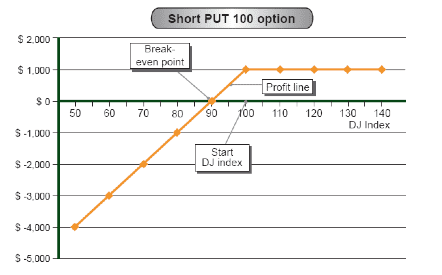# Strategy Number 4 – Short Put Option

 Market dATA DJ Index 100 points Prices Put 100 \$1,000

Strategy name:

Short Put option

Recommended use of strategy

Expectation of increase in DJ Index

Strategy components

Writing a Put option

Example: Short Put 100 at a price of \$1,000

Expenses / Income from building the strategy (at start date)

Income of \$1,000

Strategy graph:Auxiliary table for building the profit line

 DJ Index (Horizontal axis) (Fixed expense) / fixed income Variable expenses (Put contribution) Variable income Total profit / (loss) (Vertical axis) 2+3+4      50 \$1,000 (\$5,000) – (\$4,000) 60 \$1,000 (\$4,000) – (\$3,000) 70 \$1,000 (\$3,000) – (\$2,000) 80 \$1,000 (\$2,000) – (\$1,000) 90 \$1,000 (\$1,000) – \$0 100 \$1,000 – – \$1,000 110 \$1,000 – – \$1,000 120 \$1,000 – – \$1,000 130 \$1,000 – – \$1,000 140 \$1,000 – – \$1,000

Strategy analysis:

Source of loss

Loss arising from the Put option (when the DJ Index declines below the exercise index of 100 points). The loss increases as the index falls.

Source of profit

The profit arising from building the strategy – \$1,000.

Break-even point

When the loss from the option reaches the income from writing the option (\$1,000). This occurs at index 90.